Sie sind hier: ICP » R. Hilfer » Publikationen

# 1 Introduction and formulation of the problem

[1.1.1.1] A predictive macroscopic theory of two phase flow inside a rigid porous medium is of fundamental importance for many applied sciences such as hydrology, catalysis, petrophysics or filtration technology [22, 24, 5, 1, 18, 8, 13, 12]. [1.1.1.2] Despite being used in innumerable physics and engineering applications the accepted theory (see below) based on capillary pressure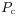and relative permeability functions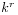lacks a sound theoretical foundation, and its basic parameter functionsandare difficult to measure in experiment [1, 21, 8].

[1.1.2.1] Modern investigations often abandon the traditional theory and resort to microscopic models (e.g. network models) as an alternative to predict macroscopic immiscible displacement in porous media [7, 9, 6, 3, 2, 10]. [1.1.2.2] An important motivation for these alternative investigations are the unresolved problems with the traditional macroscopic theory based on capillary pressure and relative permeabilities and the necessity to relate these functions to pore scale parameters. [1.1.2.3] It is therefore adequate to remind the reader of the traditional theory, introduced more than 60 years ago [28, 23, 19], and its problems. [1.1.2.4] One formulation of the traditional theory starts from the fundamental balance laws of continuum mechanics for two fluids (called water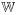and oil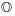) inside the pore space (called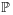) of a porous sample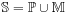with a rigid solid matrix (called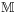). [1.1.2.5] Recall the law of mass balance in differential form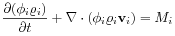(1)

where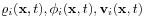denote mass density, volume fraction and velocity of phase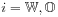as functions of position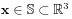and time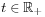. [1.1.2.6] Exchange of mass between the two phases is described by mass transfer rates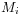giving the amount of mass by which phase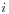changes per unit time and volume. [1.1.2.7] Momentum balance for the two fluids requires in addition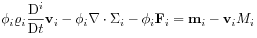(2)

where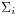is the stress tensor in theth phase,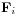is the body force per unit volume acting on theth phase,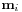is the momentum transfer into phasefrom all the other phases, and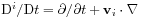denotes the material derivative for phase.

[1.2.1.1] Defining the saturations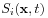as the volume fraction of pore spacefilled with phaseone has the relation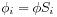where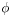is the porosity of the sample. [1.2.1.2] Expressing volume conservation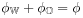in terms of saturations yields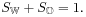(3)

[1.2.1.3] In order to get the traditional theory these balance laws for mass, momentum and volume have to be combined with specific constitutive assumptions for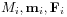and.

[1.2.2.1] Great simplification is afforded by assuming that the porous medium is macroscopically homogeneous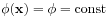(4)

although this assumption can be relaxed, and is rarely valid in practice . [1.2.2.2] Let us further assume that the fluids are incompressible so that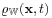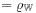(5a)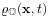(5b)

where the constants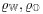are independent of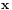and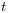. [1.2.2.3] One assumes next that the stress tensor of the fluids is diagonal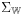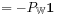(6a)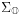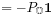(6b)

where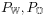are the fluid pressures. [1.2.2.4] Realistic subsurface flows have low Reynolds numbers so that the inertial term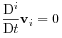(7)

[page 2, §0]   can be neglected in the momentum balance equation (2). [2.0.0.1] It is further assumed that the body forces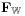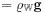(8a)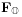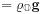(8b)

are given by gravity. [2.0.0.2] As long as there are no chemical reactions between the fluids the mass transfer rates vanish, so that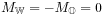(9)

holds. [2.0.0.3] Momentum transfer between the fluids and the rigid matrix is governed by viscous drag in the form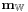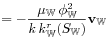(10a)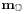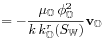(10b)

where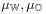are the constant fluid viscosities,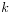is the absolute permeability, and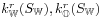are the so called relative permeabilities of water and oil.

[2.0.1.1] Inserting the constitutive assumptions (4)–(10) into the mass balance eqs. (1) yields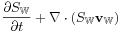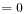(11a)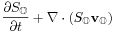(11b)

while the momentum balance eqs. (2)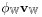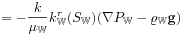(12a)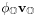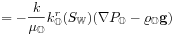(12b)

give the generalized Darcy laws for the Darcy velocities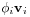[24, p. 155]. [2.0.1.2] The relative permeabilities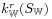,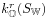are assumed to be nonlinear constitutive parameter functions. [2.0.1.3] They account for the fact, that the permability of the two immiscible fluids deviates from their partial permeabilities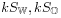obtained from volume averaging of the absolute permeability.

[2.0.2.1] Equations (11) and (12) together with eq. (3) provide 9 equations for 10 unknowns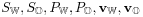. [2.0.2.2] An additional equation is needed. [2.0.2.3] Based on the work of Smith on capillary rise in regular packings  it was argued in Ref.  that the pressure difference between oil and water should in general depend only on saturation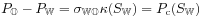(13)

where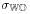is the oil-water interfacial tension and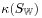is the mean curvature of the oil-water interface. [2.0.2.4] This assumption has remained the cornerstone of the theory of macroscopic capillarity for 60 years, and it is being challenged here. [2.1.0.1] The nonlinear constitutive parameter function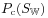is called the capillary pressure-saturation relation and it is supposed to describe the macroscopic effect of capillarity in hydrostatic equilibrium (without flow). [2.1.0.2] In practice the-curve is often parametrized in the so called van-Genuchten parametrization by five parameters consisting of two limiting saturations, two exponents and one pressure prefactor . [2.1.0.3] The traditional theory requires 5+4+3=12 parameters to parametrize a bounding hysteresis loop consisting of two drainage and one imbibition curve.

[2.1.1.1] This concludes my presentation of the traditional theory. [2.1.1.2] Equations (3),(11),(12) and (13) appropriately supplemented with initial and boundary conditions constitute the traditional theory of macroscopic capillarity in porous media. [2.1.1.3] In numerous engineering applications eqs.(3),(11),(12) and (13) are solved by computer programs .

[2.1.2.1] Serious problems limit the predictive power of equations (3),(11), (12) and (13). [2.1.2.2] The biggest problem arises from eq. (13), because it is not unique and does not account for residual saturations. [2.1.2.3] It is tacitly assumed that fluids trapped in pendular rings, ganglia or blobs behave in the same way as fluids that percolate to the sample surface. [2.1.2.4] Moreover, combining eq. (13) with eq. (12) implies that in static equilibrium, when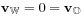, the pressure is everywhere hydrostatic, even in the trapped fluids. [2.1.2.5] This is clearly not the case.

[2.1.3.1] Other problems with,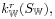and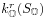are multivaluedness, hysteresis, and dynamic effects. [2.1.3.2] Equation (13) is also problematic because it seems to identify a pressure defined on the pore scale with a macroscopically averaged pressure.

[2.1.4.1] Most practitioners ignore these problems and continue to use the traditional set of equations. [2.1.4.2] Many physicists on the other hand try to overcome these problems by resorting to microscopic model calculations in an attempt to predict macroscopic behaviour starting from the pore scale or below [7, 20]. [2.1.4.3] My objective in this paper is to present a purely macroscopic approach in the same spirit as the traditional theory, but without requiring capillary pressureor relative permeabilities as input parameters. [2.1.4.4] The approach followed here is to modify the constitutive assumptions (4)–(10) based on the insight that the main effect of capillarity is the distinction between percolating and nonpercolating (trapped) fluid phases [14, 16, 15]. [2.1.4.5] The fluids flow hydrodynamically in the percolating regions while the trapped fluids are kept in place by capillary forces. Trapped fluid can only move by viscous drag or through coalescence with percolating fluid regions.

[2.1.5.1] Before defining percolating and nonpercolating fluid phases in the next section, it is appropriate to comment on the relation to other approaches. [2.1.5.2] Firstly, several authors (including the present one) have emphasized the importance of introducing the fluid-fluid surface area as a state variable (see [14, 16, 15] and references therein). [2.1.5.3] The present approach differs from such theories. [2.1.5.4] The present theory is based only on volume fractions. [2.1.5.5] It avoids surface area and its concomitant proliferation of unknowns and constitutive relations. [2.1.5.6] Secondly, some models generalize eq. (13) into a so called dynamic capillary pressure by including a dependence on rates of saturation change (see e.g.  and references therein). [2.1.5.7] The present approach includes dynamic (or viscous) effects on capillarity [page 3, §0]   in a more fundamental way by avoiding the concept of capillary pressure. [3.0.0.1] Thirdly, there exist several ad-hoc models for hysteresis loops based on rescaling the main loop formed by the bounding drainage and imbibition curves (see e.g.  and references therein). [3.0.0.2] Again, such approaches differ fundamentally from the present one, because they are based on the traditional concepts of capillary pressure and relative peremeability, while the present approach challenges the basis of these concepts. [3.0.0.3] It will be seen below that the present theory requires fewer parameters than previous theories.